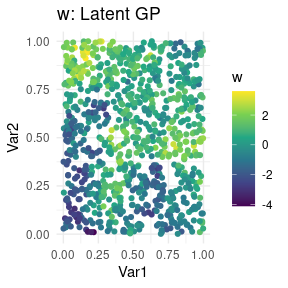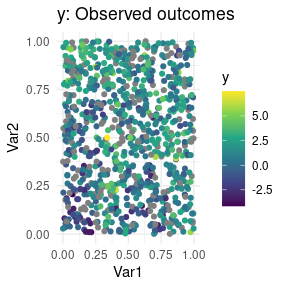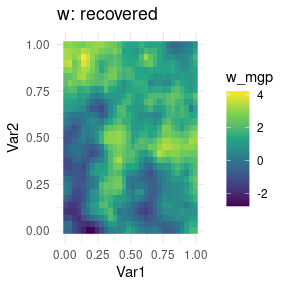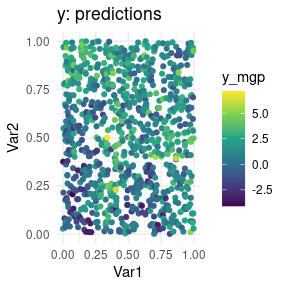# MGPs for univariate data at irregularly spaced locations

#### 2021-10-06

Compared to the univariate gridded Gaussian case, we now place the data irregularly and assume we observe counts rather than a Gaussian response.

library(magrittr)
library(dplyr)
library(ggplot2)
library(meshed)
set.seed(2021)

SS <- 30 # coord values for jth dimension
dd <- 2 # spatial dimension
n <- SS^2 # number of locations
p <- 3 # number of covariates

# irregularly spaced
coords <- cbind(runif(n), runif(n))
colnames(coords) <- c("Var1", "Var2")

sigmasq <- 1.5
nu <- 0.5
phi <- 10
tausq <- .1
ee <- rnorm(n) * tausq^.5

# covariance at coordinates
CC <- sigmasq * exp(-phi * as.matrix(dist(coords)))
# cholesky of covariance matrix
LC <- t(chol(CC))
# spatial latent effects are a GP
w <- LC %*% rnorm(n)

# covariates and coefficients
X <- matrix(rnorm(n*p), ncol=p)
Beta <- matrix(rnorm(p), ncol=1)

# univariate outcome, fully observed
y_full <- 1 + X %*% Beta + w + ee

# now introduce some NA values in the outcomes
y <- y_full %>% matrix(ncol=1)
y[sample(1:n, n/5, replace=FALSE), ] <- NA

simdata <- coords %>%
cbind(data.frame(Outcome_full= y_full,
Outcome_obs = y,
w = w))

simdata %>% ggplot(aes(Var1, Var2, color=w)) +
geom_point() +
scale_color_viridis_c() +
theme_minimal() + ggtitle("w: Latent GP")simdata %>% ggplot(aes(Var1, Var2, color=y)) +
geom_point() +
scale_color_viridis_c() +
theme_minimal() + ggtitle("y: Observed outcomes")In spmeshed we can choose settings$forced_grid=FALSE, in which case we are fitting the model $y = X \beta + w + \epsilon,$ where $$w \sim MGP$$ are spatial random effects and $$\epsilon \sim N(0, \tau^2 I_n)$$. If instead we choose settings$forced_grid=TRUE, then the model becomes $y = X \beta + H w + \epsilon',$ where $$w$$ are now sampled on a grid of knots, $$H$$ is a matrix that interpolates $$w$$ to the observed locations, and $$\epsilon \sim N(0, R + \tau^2 I_n)$$ where $$R$$ is a diagonal matrix that corrects the measurement error variance. Refer to Peruzzi et al (2021)1 for details.

Regardless of the model chosen, for spatial data, an exponential covariance function is used by default: $$C(h) = \sigma^2 \exp( -\phi h )$$ where $$h$$ is the spatial distance.

The prior for the spatial decay $$\phi$$ is $$U[l,u]$$ and the values of $$l$$ and $$u$$ must be specified. We choose $$l=1$$, $$u=30$$ for this dataset.2

Setting verbose tells spmeshed how many intermediate messages to output while running MCMC. We set up MCMC to run for 3000 iterations, of which we discard the first third. We use the argument block_size=25 to specify the coarseness of domain partitioning. In this case, the same result can be achieved by setting axis_partition=c(10, 10).

Finally, we note that NA values for the outcome are OK since the full dataset is on a grid. spmeshed will figure this out and use settings optimized for partly observed lattices, and automatically predict the outcomes at missing locations. On the other hand, X values are assumed to not be missing.

mcmc_keep <- 200 # too small! this is just a vignette.
mcmc_burn <- 400
mcmc_thin <- 2

mesh1_total_time <- system.time({
meshout1 <- spmeshed(y, X, coords,
block_size = 25,
n_samples = mcmc_keep, n_burn = mcmc_burn, n_thin = mcmc_thin,
verbose = 0,
settings=list(forced_grid=FALSE, cache=FALSE),
prior=list(phi=c(1,30))
)})
#> Warning in spmeshed(y, X, coords, block_size = 25, n_samples = mcmc_keep, : Data
#> look not gridded: force a grid with settings$forced_grid=T. And now with the gridded knots. We build a grid with as many knots as there are observations, but we could use a smaller or a bigger one. mesh2_total_time <- system.time({ meshout2 <- spmeshed(y, X, coords, grid_size = c(30, 30), block_size = 25, n_samples = mcmc_keep, n_burn = mcmc_burn, n_thin = mcmc_thin, n_threads = 2, verbose = 0, prior=list(phi=c(1,30)) )}) Post-processing proceeds the same way as when the data locations are on a grid. Let’s do that for the second model. summary(meshout2$lambda_mcmc[1,1,]^2)
#>    Min. 1st Qu.  Median    Mean 3rd Qu.    Max.
#>   1.425   2.286   2.767   3.174   3.628   7.606
summary(meshout2$theta_mcmc[1,1,]) #> Min. 1st Qu. Median Mean 3rd Qu. Max. #> 1.138 2.471 3.386 3.261 3.900 6.171 summary(meshout2$tausq_mcmc[1,])
#>    Min. 1st Qu.  Median    Mean 3rd Qu.    Max.
#> 0.07923 0.15122 0.16977 0.17221 0.19697 0.26921
summary(meshout2$beta_mcmc[1,1,]) #> Min. 1st Qu. Median Mean 3rd Qu. Max. #> -1.403 -1.345 -1.327 -1.328 -1.312 -1.261 We proceed to plot predictions across the domain along with the recovered latent effects. We plot the predictions at the input locations, i.e. the points not on the grid of knots, which we find via forced_grid==0. We plot the recovered latent process on the grid, for a nicer picture. # process means wmesh <- data.frame(w_mgp = meshout2$w_mcmc %>% summary_list_mean())
# predictions
ymesh <- data.frame(y_mgp = meshout2$yhat_mcmc %>% summary_list_mean()) outdf <- meshout2$coordsdata %>%
cbind(wmesh, ymesh) %>%
left_join(simdata)

outdf %>% filter(forced_grid==1) %>%
ggplot(aes(Var1, Var2, fill=w_mgp)) +
geom_raster() +
scale_fill_viridis_c() +
theme_minimal() + ggtitle("w: recovered")outdf %>% filter(forced_grid==0) %>%
ggplot(aes(Var1, Var2, color=y_mgp)) +
geom_point() +
scale_color_viridis_c() +
theme_minimal() + ggtitle("y: predictions")1. spmeshed implements a model which can be interpreted as assigning $$\sigma^2$$ a folded-t via parameter expansion if settings$ps=TRUE, or an inverse Gamma with parameters $$a=2$$ and $$b=1$$ if settings$ps=FALSE, which cannot be changed at this time. $$\tau^2$$ is assigned an exponential prior.↩︎